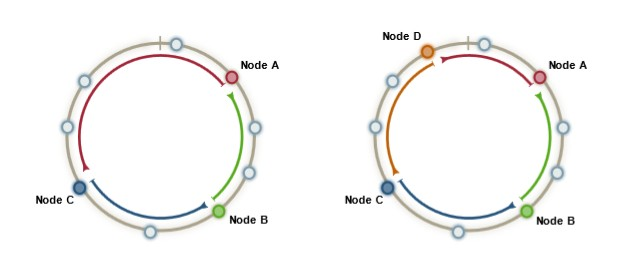# Cryptography - Input Data (plain text | clear text | message)

Plain text is a text that you want to send.

Plain text is also known as

• Cleartext
• Usable text
• Message

Even if its name contains text, the input can be any piece of data:

## Operation

### Encryption

You can give a message as input to an cipher function (encryption) that outputs a ciphertext

### Signature / Data Integrity

You can give a message as input to an cipher function (encryption) that outputs a hash

Recommended PagesAuthenticity

is a functionality of cryptography that verifies that the sender of the message is who he claimed to be. This functionality is implemented with the digital signature functionality. And as a side effect,...Padding fill the last block of a file to be processed by a block cipher. For the , , and modes, the plaintext must be a sequence of one or more complete data blocks (or, for CFB mode, data segments)....Crypto

graphy is the science of cryptographic algorithms (known as Cipher) that implements the following functionalities: Data Integrity - the message (data) was not altered during transit Authenticity -...Cryptography - (Ciphertext|cyphertext|Message Digest|Digest)

In cryptography, ciphertext or cyphertext is the result of encryption performed on plaintext using an algorithm, called a cipher. In the context of hashing / data integrity, the cyphertext is known...Cryptography - Algorithm (called a Cipher)

A cipher is an algorithm that encrypt a plain text message into a ciphertext. Cipher algorithm can be categorized by the key that they used as parameters. No key symmetric one (using one key) ...Cryptography - Base64 Cipher

Base64 is a cipher (without key and reversible) that converts (encode) a data (message) in 64 characters of the ASCII string. See also the Google...Cryptography - Decryption (Deciphering)

A process from a cipher that transforms encrypted data into the original usable dataCryptography - Encryption (Enciphering)

Encryption is the function of a cipher that transforms usable data into an unreadable form. It provides confidentiality if a secret is used in the encryption. There is two class of encryption. ...Cryptography - Hash

A hash function is an encryption crypto algorithm that takes as data as input (possibly large and of variable-sized) and produces a short fixed-length integer value (generally printed as an hexadecimal...Cryptography - Key

A key is a parameter used in a cipher algorithm that determines the encryption operation (forward) and the decryption operation (backward). It's the only secret parameter that protect the anonymity...